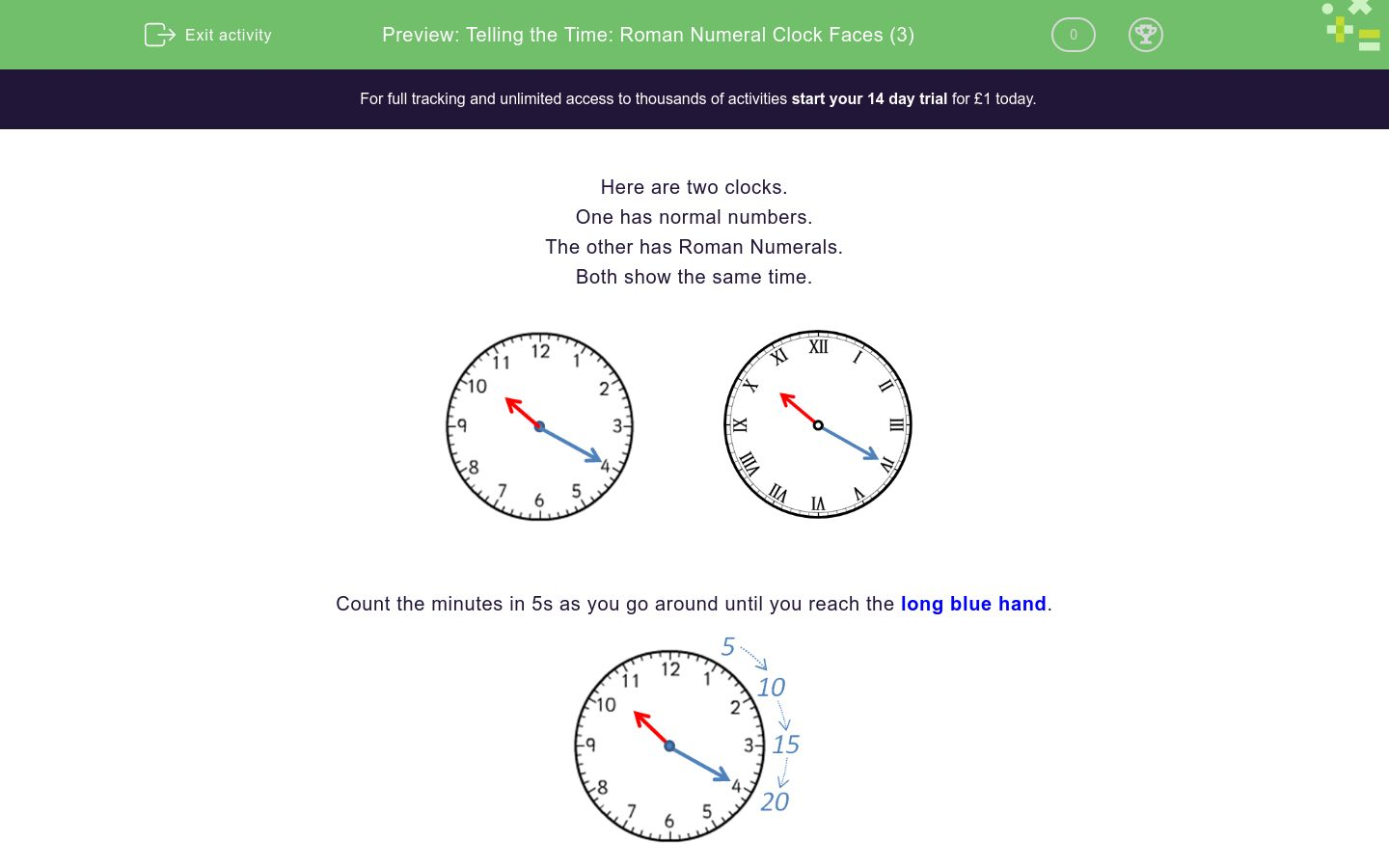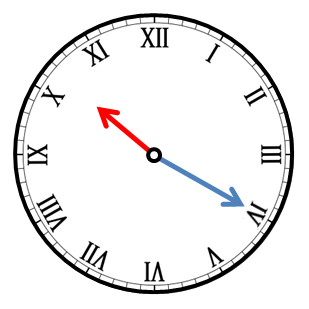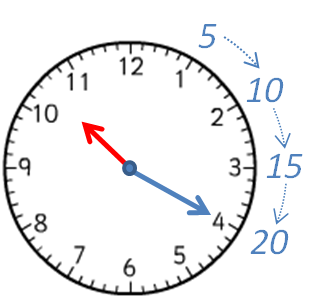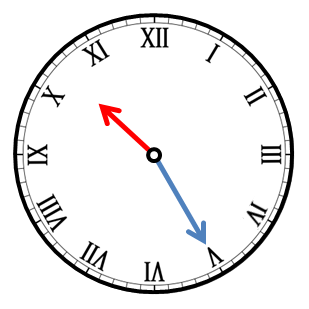# Telling the Time: Roman Numeral Clock Faces (3)

In this worksheet, students must select the time that matches the given Roman Numeral clock face. Times in multiples of 5 minutes are shown.Key stage:  KS 2

Curriculum topic:   Measurement

Curriculum subtopic:   Tell the Time (Roman Numerals from I to XII)

Difficulty level:### QUESTION 1 of 10

Here are two clocks.

One has normal numbers.

The other has Roman Numerals.

Both show the same time.Count the minutes in 5s as you go around until you reach the long blue hand.The long blue hand is pointing to the 4 or IV, which is 4 × 5 = 20 minutes, so the time will be something  20.

The short red hand is pointing between the 10 and the 11 or the X and the XI, so the time is:

10:20

or 20 past 10 because it is 20 minutes past 10 o'clock.

Here are two more clocks, showing the same time.Count the minutes in 5s as you go around until you reach the long blue hand.The long blue hand is pointing to the 7 or the VII, which is 7 × 5 = 35 minutes, so the time will be something  35.

The short red hand is pointing between the 2 and the 3 or the II and the III, so the time is:

2:35

or 25 to 3 because there are 25 mins (minutes) until 3 o'clock.

Which time is shown on the clock?10:25

10:05

10:15

Which time is shown on the clock?2:15

3:10

Which time is shown on the clock?11:08

11:40

12:40

Which time is shown on the clock?7:11

5 past 8

5 to 8

7:55

Write the time shown on the clock.

Use a colon : to separate the hour from the minutes (e.g. 7:30).Write the time shown on the clock.

Use a colon : to separate the hour from the minutes (e.g. 7:30).Write the time shown on the clock.

Use a colon : to separate the hour from the minutes (e.g. 7:30).Which time is shown on the clock?5 to 4

4:55

5:55

5 to 5

Which time is shown on the clock?20 to 8

20 to 7

8 to 8

Which time is shown on the clock?10 to 1

10 past 12

2 past 12

• Question 1

Which time is shown on the clock?10:25
EDDIE SAYS
The short hand is pointing to between the 10 and the 11.
The long hand is pointing to 5, which is 25 mins.
• Question 2

Which time is shown on the clock?3:10
EDDIE SAYS
The short hand is pointing to between the 3 and the 4.
The long hand is pointing to 2, which is 10 mins.
• Question 3

Which time is shown on the clock?11:40
EDDIE SAYS
The short hand is pointing to between the 11 and the 12.
The long hand is pointing to 8, which is 40 mins.
• Question 4

Which time is shown on the clock?5 to 8
7:55
EDDIE SAYS
The short hand is pointing to between the 7 and the 8.
The long hand is pointing to 11, which is 55 mins or '5 to'.
• Question 5

Write the time shown on the clock.

Use a colon : to separate the hour from the minutes (e.g. 7:30).11:05
EDDIE SAYS
The short hand is pointing to between the 11 and the 12.
The long hand is pointing to 1, which is 5 mins.
• Question 6

Write the time shown on the clock.

Use a colon : to separate the hour from the minutes (e.g. 7:30).01:05
1:05
EDDIE SAYS
The short hand is pointing to between the 1 and the 2.
The long hand is pointing to 1, which is 5 mins.
• Question 7

Write the time shown on the clock.

Use a colon : to separate the hour from the minutes (e.g. 7:30).01:25
1:25
EDDIE SAYS
The short hand is pointing to between the 1 and the 2.
The long hand is pointing to 5, which is 25 mins.
• Question 8

Which time is shown on the clock?4:55
5 to 5
EDDIE SAYS
The short hand is pointing to between the 4 and the 5.
The long hand is pointing to 11, which is 55 mins or '5 to'.
• Question 9

Which time is shown on the clock?20 to 8
EDDIE SAYS
The short hand is pointing to between the 7 and the 8.
The long hand is pointing to 8, which is '20 to' or 40 mins past.
• Question 10

Which time is shown on the clock?10 past 12
EDDIE SAYS
The short hand is pointing to between the 12 and the 1.
The long hand is pointing to 2, which is 10 mins past.
---- OR ----

Sign up for a £1 trial so you can track and measure your child's progress on this activity.

### What is EdPlace?

We're your National Curriculum aligned online education content provider helping each child succeed in English, maths and science from year 1 to GCSE. With an EdPlace account you’ll be able to track and measure progress, helping each child achieve their best. We build confidence and attainment by personalising each child’s learning at a level that suits them.

Get started